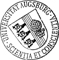## Projection Based Model Reduction for Optimal Design of the Time-Dependent Stokes System

• The optimal design of structures and systems described by partial differential equations (PDEs) often gives rise to large-scale optimization problems, in particular if the underlying system of PDEs represents a multi-scale, multi-physics problem. Therefore, reduced order modeling techniques such as balanced truncation model reduction, proper orthogonal decomposition, or reduced basis methods are used to significantly decrease the computational complexity while maintaining the desired accuracy of the approximation. In particular, we are interested in such shape optimization problems where the design issue is restricted to a relatively small portion of the computational domain. In this case, it appears to be natural to rely on a full order model only in that specific part of the domain and to use a reduced order model elsewhere. A convenient methodology to realize this idea consists in a suitable combination of domain decomposition techniques and balanced truncation model reduction. WeThe optimal design of structures and systems described by partial differential equations (PDEs) often gives rise to large-scale optimization problems, in particular if the underlying system of PDEs represents a multi-scale, multi-physics problem. Therefore, reduced order modeling techniques such as balanced truncation model reduction, proper orthogonal decomposition, or reduced basis methods are used to significantly decrease the computational complexity while maintaining the desired accuracy of the approximation. In particular, we are interested in such shape optimization problems where the design issue is restricted to a relatively small portion of the computational domain. In this case, it appears to be natural to rely on a full order model only in that specific part of the domain and to use a reduced order model elsewhere. A convenient methodology to realize this idea consists in a suitable combination of domain decomposition techniques and balanced truncation model reduction. We will consider such an approach for shape optimization problems associated with the time-dependent Stokes system and derive explicit error bounds for the modeling error. As an application in life sciences, we will be concerned with the optimal design of capillary barriers as part of a network of microchannels and reservoirs on microfluidic biochips that are used in clinical diagnostics, pharmacology, and forensics for high-throughput screening and hybridization in genomics and protein profiling in proteomics.• Dokument_1.pdfAuthor: Thomas FrankeGND, Ronald H. W. HoppeGND, Christopher Linsenmann, Achim WixforthORCiDGND urn:nbn:de:bvb:384-opus4-11342 https://opus.bibliothek.uni-augsburg.de/opus4/1354 Preprints des Instituts für Mathematik der Universität Augsburg (2010-02) Preprint English Universität Augsburg University of Houston 2010/02/03 projection based model reduction; shape optimization; time-dependent Stokes system; domain decomposition; balanced truncation; biochips Gestaltoptimierung; Partielle Differentialgleichung; Gebietszerlegungsmethode; Mathematisches Modell; Ordnungsreduktion; Biochip; Mikrofluidik Mathematisch-Naturwissenschaftlich-Technische Fakultät Mathematisch-Naturwissenschaftlich-Technische Fakultät / Institut für Mathematik Mathematisch-Naturwissenschaftlich-Technische Fakultät / Institut für Physik Mathematisch-Naturwissenschaftlich-Technische Fakultät / Institut für Mathematik / Lehrstuhl für Numerische Mathematik Mathematisch-Naturwissenschaftlich-Technische Fakultät / Institut für Physik / Lehrstuhl für Experimentalphysik I 5 Naturwissenschaften und Mathematik / 51 Mathematik / 510 MathematikDeutsches Urheberrecht mit Print on Demand